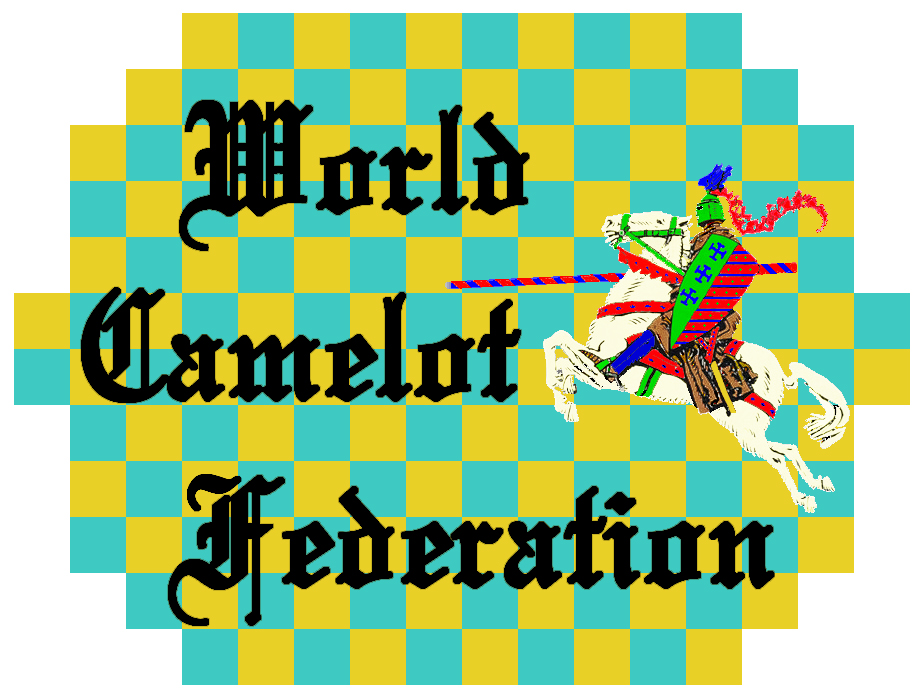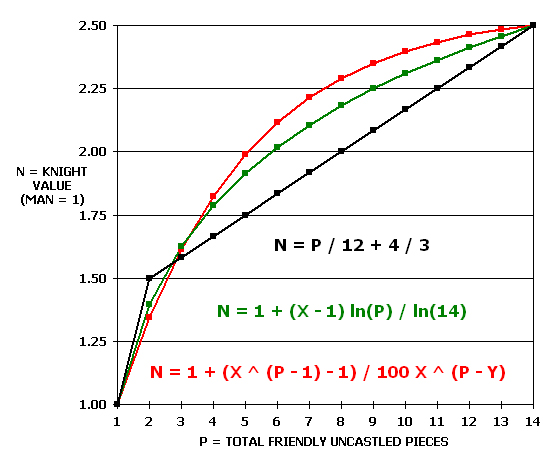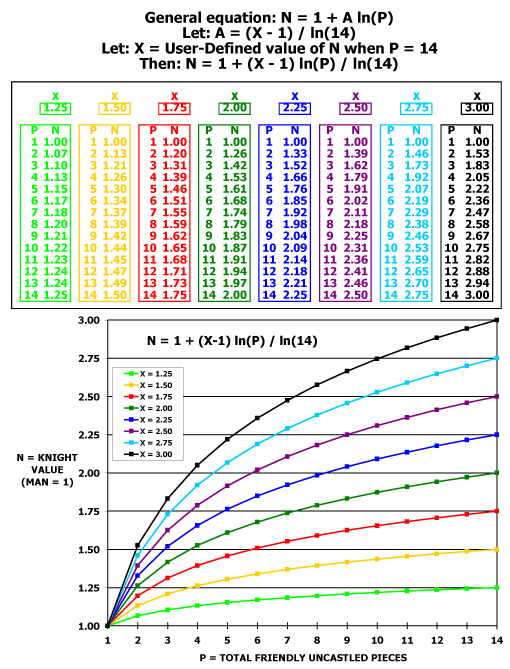KNIGHT-MAN VALUATION   Although Camelot is a unique game, it shares many of its conceptual characteristics with several other games.  However, there is one way in which Camelot is unlike most other abstract strategy board games: as the game progresses, the determination of whether or not one side has a material advantage over the other can, at times, be rather difficult.  Certainly, the exact quantification of material advantage, when it involves differing forces of Knights and Men, is nearly impossible.  The reason for this is that the relative value of a Knight vs. the value of a Man is not only unclear at the beginning of the game; it also changes throughout the game.   This is the single most important tactical issue in Camelot: how much more than a Man is a Knight worth?  At the beginning of the game, a Knight is clearly worth more than a Man.  After all, unlike a Man, a Knight has the dynamic power to make a Knight's Charge.  But how much more than a Man is a Knight worth at the start of a game?   Just as obvious is the fact that as the game progresses and pieces are removed from the board, the value of a Knight decreases relative to the value of a Man.  The ultimate expression of this is that a solitary Knight, without supporting pieces, is worth no more than a Man.  But how fast does the value of a Knight decrease as the pieces come off the board?   The first attempted quantification of the value of a Knight resulted in the derivation of the following formula: Let: Value of a Man = 1 Let: Value of a Knight = N Let: Number of friendly uncastled pieces = P Then: If P = 1, N = 1 and if P > 1, N = P / 12 + 4 / 3   The next quantification resulted in the following formula: Let: Value of a Man = 1 Let: Value of a Knight = N Let: Number of friendly uncastled pieces = P Let: User-defined variable #1 = X Let: User-defined value #2 = Y Then: N = 1 + (X ^ (P - 1) - 1)/ 100 X ^ (P - Y)   The final quantification resulted in the following (current) formula: Let: Value of a Man = 1 Let: Value of a Knight = N Let: Number of friendly uncastled pieces = P Let: User-defined variable equal to the desired N value at the start of the game = X Then: N = 1 + (X -1) ln(P) / ln(14)   The following chart displays a comparison of the three formulae, that is, the evolution of the Knight-Man Valuation formula.The following chart displays the current formula, with eight different (user-defined) X values.  The correct X value (the value of a Knight relative to the value of a Man at the beginning of a game) is still to be determined.   It is the World Camelot's position that the value is approximately 2.25, but the validity of that opinion will be extensively tested using Chaxx, the world champion computer program.Obviously, all of this theory depends not only upon the correctness of the formula's derivation from a statistical standpoint (is the X value correct?), but also from a conceptual standpoint (is the formula in the correct mathematical form to properly express changing N values?).   In any case, to put all of this in perspective, the correct value of Knight vs. Man will determine how Camelot should be played, in the opening, in the the middlegame, and in the endgame.   One final point; in Chess, a player who wins a Rook for a Bishop or a Rook for a Knight is said to have "won the exchange."  To borrow Chess terminology, and coin a new Camelot term, we will define winning the exchange in Camelot as the winning of a Knight for a Man.  In Chess, given an otherwise even position, winning the exchange will usually win the game.  Will winning the exchange in Camelot win the game?  Because of the ever-changing value of a Knight, this is a question that cannot easily be answered.